# ISEE Upper Level Quantitative : How to factor a number

## Example Questions

← Previous 1 3 4 5

### Example Question #1 : How To Factor A Number

Which of these numbers is relatively prime with 18?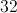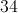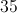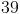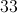Explanation:

For two numbers to be relatively prime, they cannot have any factor in common except for 1. The factors of 18 are 1, 2, 3, 6, 9, and 18.

We can eliminate 32 and 34, since each shares with 18 a factor of 2; we can also eliminate 33 and 39, since each shares with 18 a factor of 3. The factors of 35 are 1,  5, 7, and 35; as can be seen by comparing factors, 18 and 35 only have 1 as a factor, making 35 the correct choice.

### Example Question #2 : How To Factor A Number

Which of the following is the prime factorization of 333?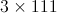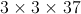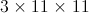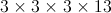333 cannot be factorized furtherExplanation:

To find the prime factorization, break the number down as a product of factors, then keep doing this until all of the factors are prime.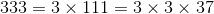### Example Question #3 : How To Factor A Number

Give the prime factorization of 91.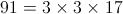91 is a prime number.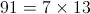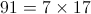Explanation: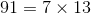Both are prime factors so this is the prime factorization.

### Example Question #4 : How To Factor A Number

How many factors does 40 have?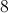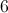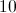Explanation:

40 has as its factors 1, 2, 4, 5, 8, 10, 20, and 40 - a total of eight factors.

### Example Question #5 : How To Factor A Number

What is the sum of all of the factors of 27?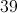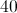Explanation:

27 has four factors: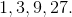Their sum is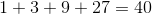.

### Example Question #6 : How To Factor A Number

Add all of the factors of 30.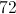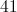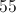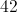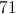Explanation:

The factors of 30 are 1, 2, 3, 5, 6, 10, 15, and 30. Their sum is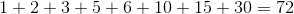.

### Example Question #7 : How To Factor A Number

Which is the greater quantity?

(a) The number of factors of 169

(b) The number of factors of 121

(b) is greater

(a) is greater

It is impossible to tell from the information given

(a) and (b) are equal

(a) and (b) are equal

Explanation:

Each number has only three factors. 121 has 1, 11, and 121 as factors; 169 has 1, 13, and 169 as factors. The answer is that the quantities are equal.

### Example Question #8 : How To Factor A Number

Which is the greater quantity?

(a) The number of factors of 15

(b) The number of factors of 17

(a) is greater.

It is impossible to tell from the information given.

(b) is greater.

(a) and (b) are equal.

(a) is greater.

Explanation:

(a) 15 has four factors, 1, 3, 5, and 15.

(b) 17, as a prime, has two factors, 1 and 17.

Therefore, (a) is greater.

### Example Question #9 : How To Factor A Number

Which is the greater quantity?

(a) The product of the integers between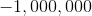and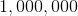inclusive

(b) The sum of the integers betweenandinclusive

(b) is greater.

It is impossible to tell from the information given.

(a) and (b) are equal.

(a) is greater.

(a) and (b) are equal.

Explanation:

The quanitites are equal, as both can be demonstrated to be equal to.

(a) One of the integers in the given range is, so one of the factors will be, making the product.

(b) The sum of the numbers will be: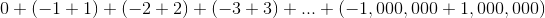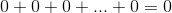### Example Question #10 : How To Factor A Number

Which is the greater quantity?

(a) The sum of the factors of(b) The sum of the factors of(a) and (b) are equal.

It is impossible to tell from the information given.

(a) is greater.

(b) is greater.

(b) is greater.

Explanation:

(a) The factors ofare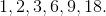Their sum is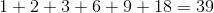.

(b) The factors ofare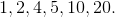Their sum is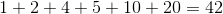.

(b) is greater.

← Previous 1 3 4 5